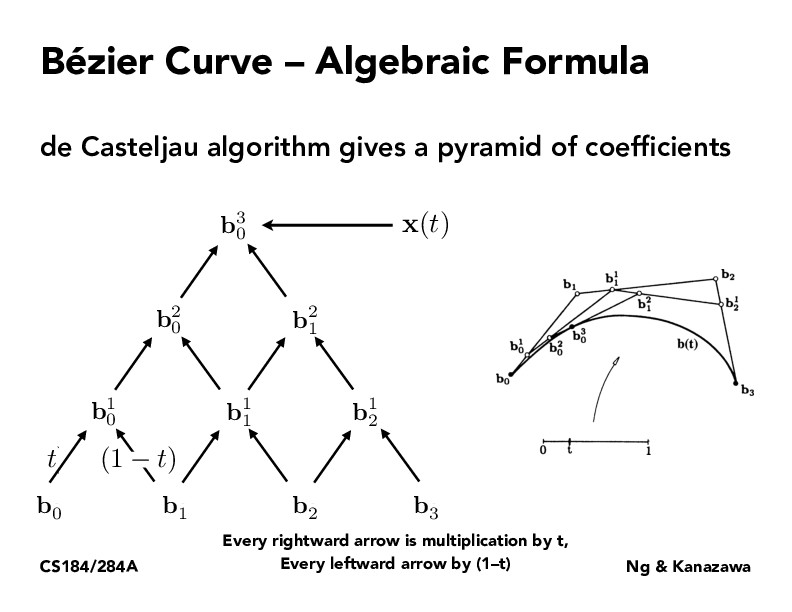Lecture 7: Splines and Bezier Curves (68)mie-h

Just to clarify, are we trying to find a curve to fit the points b0,b1,b2,b3, given a t value? And every other point was determined recursively to help us fit that curve? And what is the difference between starting with three points (like in the last side) and starting with 4 dots, like shown here? Is there a reason we would start with 4, instead of 5?andywu1000

Yeah the 4 points, $b_0, b_1, b_2, b_3$ are the control points used to generate the bezier curve. Then, as you said you recursively lerp $b_0$ and $b_1$ to get $b_0^1$ and so on until they combine into a single point, $b_0^3$, which is the curve. As you sweep $t$ between 0 and 1, the path of $b_0^3$ generates the final bezier curve. Starting with more control points gives you more control over the shape of the generated curve.

You must be enrolled in the course to comment